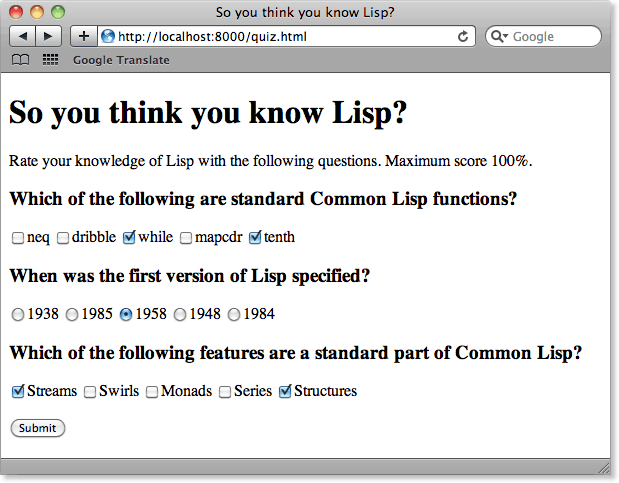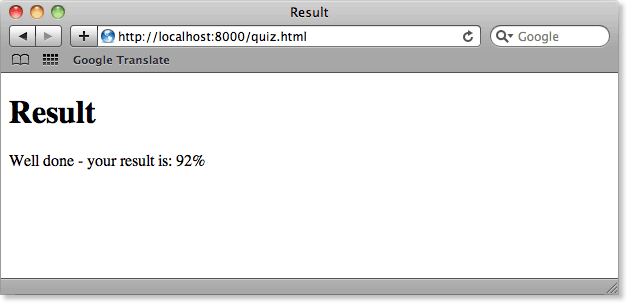## Processing Form Fields

Our next example is a more advanced use of forms to create an interactive quiz. It is based on a quiz-handling program we designed for a corporate Web site. The questions in the quiz are all coded in the quiz form itself; the response function only needs to know the score to give to each answer.

Full listing

### Description

This application illustrates how to generate checkboxes and radio-buttons using CL-HTTP, and shows how to parse the association list returned by a form.

As in the previous example we use with-fillout-form to define a form. The form includes two sets of checkboxes and a set of radio buttons.

Form fields are displayed using the CL-HTTP function accept-input. For example, a set of checkboxes is displayed with:

```(accept-input 'checkbox "Q1" :stream stream
:choices '("One" "Two" "Three" "Four"))```

This creates five checkboxes with the labels specified by the :choices strings:Each checkbox in the set has the name Q1. By default the checkboxes are formatted in a bulleted list. Alternatively the :layout option :none lays out the checkboxes in a row.

### Displaying the quiz form

The routine display-quiz draws the form:

```(defun display-quiz (url stream)
"The form function for a quiz. The form values are posted to the same URL."
(with-page (url stream "So you think you know Lisp?")
(with-fillout-form (:post url :stream stream)
(with-paragraph (:stream stream)
(write-string "Rate your knowledge of Lisp with the following questions. " stream)
(write-string "Maximum score 100%." stream))
(with-section-heading ("Which of the following are standard Common Lisp functions?"
:level 3 :stream stream)
(accept-input 'checkbox "Q1" :layout :none :stream stream
:choices '("neq" "dribble" "while" "mapcdr" "tenth")))
(with-section-heading ("When was the first version of Lisp specified?" :level 3 :stream stream)
:choices '("1938" "1985" "1958" "1948" "1984") :layout :none :stream stream ))
(with-section-heading ("Which of the following features are a standard part of Common Lisp?"
:level 3 :stream stream)
(accept-input 'checkbox "Q3" :layout :none :stream stream
:choices '("Streams" "Swirls" "Monads" "Series" "Structures")))
(with-paragraph (:stream stream)
(accept-input 'submit-button "Submit" :stream stream)))))```

It generates the following page:The response-function mark-quiz receives the posted form results and calculates the score. The response function is called with an association list associating the name of each form field with the index of each selected field, starting from "0". If there are several form fields with the same name, as in this case, the values are returned as a list:

`((:Q1 ("2" "4") T) (:Q2 "2") (:Q3 ("0" "4") T) (:SUBMIT Submit))`

The CL-HTTP macro bind-query-values assigns the value of each form field to a variable of the same name. From these the routine mark calculates the total score for each question by looking up the list of indices in a list containing the scores for each answer:

```(defun mark (values answers)
(reduce #'+ (if (listp values) values (list values))
:key #'(lambda (str) (nth (parse-integer str) answers))))
```

Here's the definition of mark-quiz

```(defun mark-quiz (url stream alist)
"The response function for the quiz. This processes the form values and displays the result."
(declare (ignore url))
(bind-query-values (q1 q2 q3) (url alist)
(let* ((total (+ (mark q1 '(-1 1 -1 -1 1))
(mark q2 '(0 0 2 0 0))
(mark q3 '(1 -1 -1 -1 1))))
(score (round (* (+ total 6) 100) 12)))
(with-page (url stream "Result")
(with-paragraph (:stream stream)
(cond
((> score 80)
(format stream "Well done - your result is: ~a%" score))
((> score 50)
(t
(format stream "Pretty poor - your result is: ~a%" score))))))))```

This processes the form and displays the score with an appropriate comment:Finally the URL for the quiz is exported using the following routine:

```(export-url "http://localhost:8000/quiz.html"
:html-computed-form
:form-function 'display-quiz
:response-function 'mark-quiz)```